# Do While LoopsThis activity extends the “Teaching Foundations of Computer Science With Python on TI-Nspire™ Technology” workshop to study additional topics from computer science with the support of the TI-Nspire™ CX II graphing calculator with Python coding technology. We will explore Do While loops, a form of post-test loop that always runs at least one time.

In this activity, we will:

• Model the Do While loop structure
• Use the break statement to exit out of a loop
• Construct and use iterative control structures, including a While loop written to behave like a post-test Do While loop

## Materials for learning

Also, you'll want to have the following handy:

• TI-Nspire™ CX II graphing calculator (with updated OS)

## Unit 4 Activity 3: The Do While Loop

A great introduction to this loop structure can be found in Unit 4, Activity 3. Start by working through that activity, then read through the following discussion of main ideas from the activity.

### Random Numbers example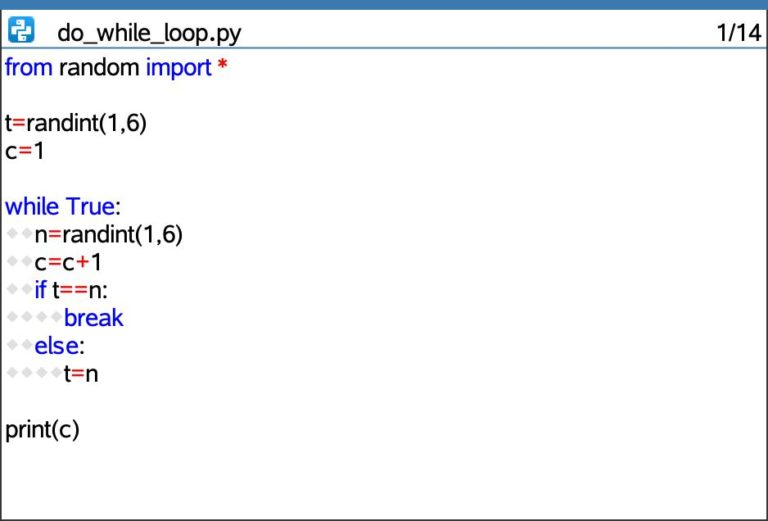Quick refresher: How does the randint(1,6) syntax work?

Answer: In Python, randint(a,b) returns a random integer within the inclusive range from a to b (meaning including a and b). So, randint(1,6) will return a random integer from the set {1, 2, 3, 4, 5, 6}.

Tech tip: randint() can be entered via the
TI-Nspire™ keyboard or from Menu > Random.

Tech tip: The break command can be typed in via the TI-Nspire™ keyboard.

How does the do_while_loop.py program at the bottom of page 1 work? This program may not be intuitive. Explain it aloud to yourself or a colleague, then read through a sample description below.

This program starts by assigning a random number from 1–6 to variable t and storing the value of 1 to variable c. c will be used as a count variable in this program to keep track of how many numbers are generated before two consecutive values are the same.

Next, a Do While loop (modeled using a While loop with a condition of True) kicks in. Within the loop, a new variable n is created and it’s assigned a random number from 1–6. Also, the value of c is increased by one to show that a new number has been randomly determined. Now c has a value of 2, representing that we have found 2 numbers so far.

Next, the program checks to see if t and n have the same value. Why? The program is checking to see if n (the new number) is the same as t (the previous number). If they are the same, that means that the program generated two consecutive numbers that are the same. If this happens, the command within the If block will run — specifically, the break command will make the program exit the While loop and move on to the print command.

If t and n don’t have the same value (what are the chances?), then the program will skip down to the criteria in the else command. In this case, the value that was stored in n (in other words, the most recent random number we found) will be stored into variable t. Now, t no longer represents the first number we found — it represents the second number we found. Why? So, that we can go on to find another, new number (our third number), store this to n, and then compare it to the number we found most recently (now stored in t).

So, what happens after the else command executes and the value of n gets saved in t? Well, we’re at the end of our loop, so the loop will circle back to its beginning. (Since the conditional for this While loop is True, it will keep running until it encounters a break command.)

The program will continue to run through this loop of finding a new random number (n), adding 1 to the count variable to keep track of how many numbers we’ve found, comparing the new number (n) to the previous number (t), and then exiting the loop if these values are the same or storing the new number to the previous number variable and continuing the process.

Then, what gets printed? The value of one of the numbers? No, c, which represents how many numbers we needed to randomly calculate before we got two in a row that were the same.

This is a great place to make some predictions:

• What do you think the minimum value of c will be? What does this represent in this context?
• What do you think the maximum value will be? What does it represent in this context?
• What numbers do you expect to see most often for c? Why? What do they represent?

### Guess My Number program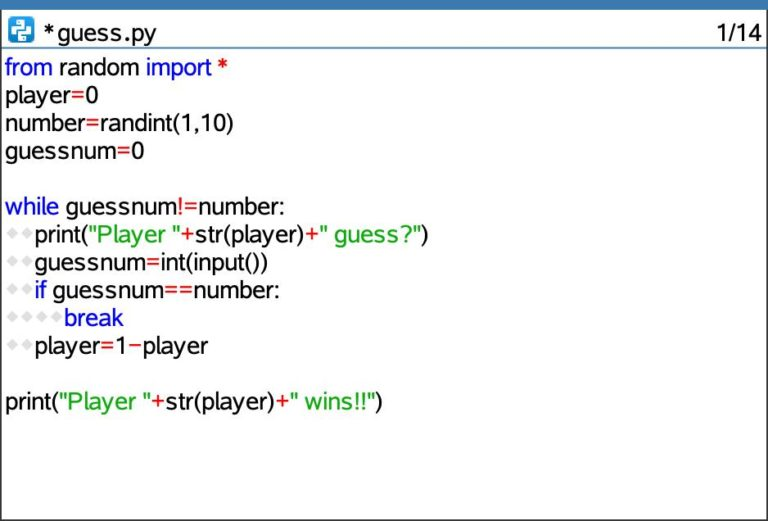How does the syntax of the print(“Player ”+str(player)+“ guess?”) line of code work? What is each part of this statement doing?

Take a minute to explain this syntax aloud to yourself or a colleague before reading the discussion below.

This line of code will print a message on the screen to the user that says Player 0 guess? or Player 1 guess? depending on whose turn it is. The program uses the variable player to keep track of the 0 and 1 values for the two players’ names.

The + symbols concatenate the various parts of the string to be printed. The str() command converts the integer value stored in the variable player into a string variable so that it can be concatenated (joined) with the other strings in the print statement.

How does the syntax of the guessnum=int(input()) line of code work? What is each part of this statement doing?

Again, take a minute to explain this to yourself first before you read the following discussion.

On the right side of the equal sign, the int(input()) command is prompting the user for input and then converting this input into an integer because numeric input is expected of the user. This value that the user enters will then be stored in variable guessnum; this is accomplished by the use of the variable name and then gets (=), the assignment operator.

Why isn’t there any text inside the parentheses of the input command? Because the instructions to the user were displayed in the print command on the line above. So, here, the input command doesn’t include any instructions and just waits for the user’s input.

Here are two extensions to try out:

• Can you condense the print(…) and guessnumber=… lines of code discussed above into one line of code? What would that look like and how would it work?
• How might the player number (in other words, Player 0 or Player 1) be displayed as Player 1 and Player 2, even though the calculator sees the player numbers as 0 and 1?

## Exploring the Do While structure

As discussed in the above activity, Python doesn’t have an explicit Do While loop structure, but we can model this structure with a specially crafted While loop. Why do this? Do While loops are powerful structures within the larger domain of computer science; it’s important to build our familiarity with these loops and how they are similar to and different than traditional While loops.

After working through that activity, consider the following loop. What do you think the output of this loop would be? How do you know?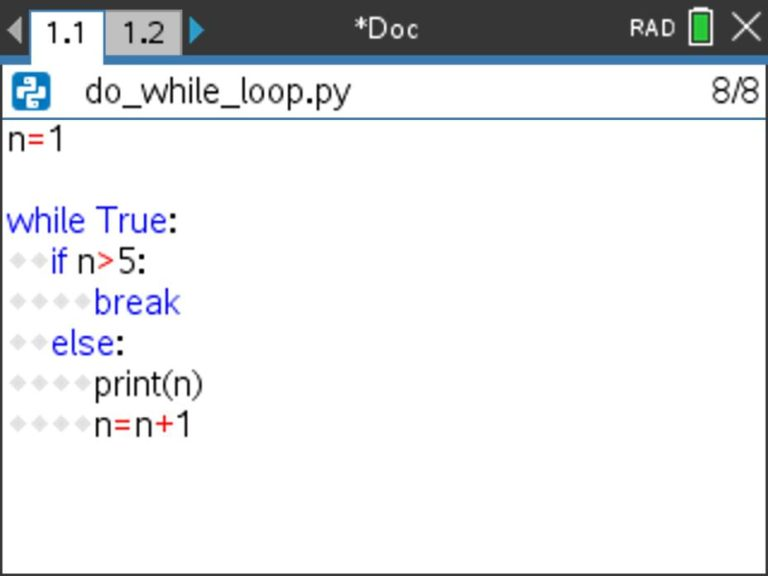Check your thinking with the output shown in the following screenshot: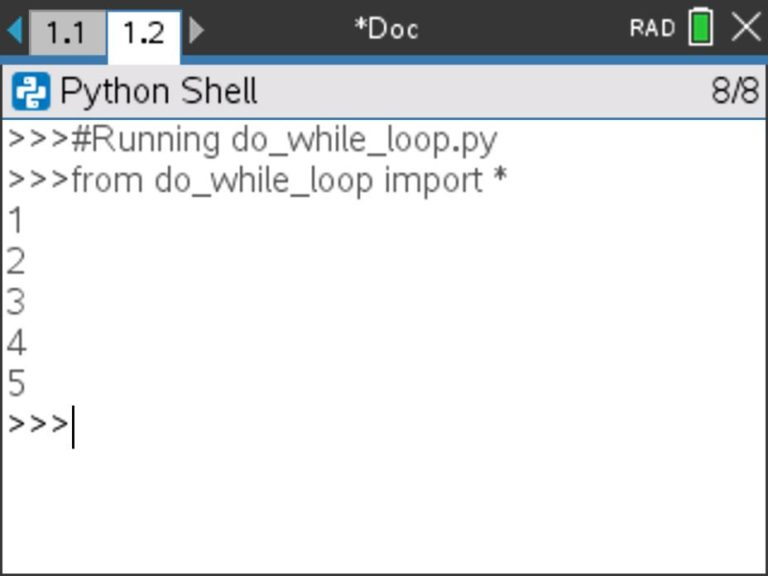How might we edit the code for this program so that the output is:

3

4

5

6

… or …

2

5

8

11

… or …

9

7

5

3

1

Take a few minutes and play around with sample code that would yield those outputs.

Side note: If you get caught in an infinite loop, don’t stress — it has happened to us all! You can exit out of an infinite loop on a handheld calculator by holding down the On/Home button for a few seconds. To exit out of an infinite loop on the TI-Nspire™ software on Windows®, press F12 then Enter repeatedly. To exit out of an infinite loop on the TI-Nspire™ software on a Mac®, press F5 then Enter repeatedly.

Here are some screenshots of sample programs that would yield the outputs shown above. How else might these programs be written using a Do While structure? These sample programs are not the only ways to achieve the desired outputs.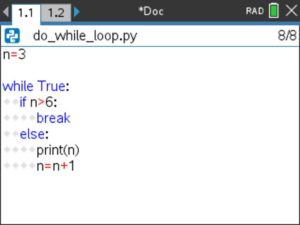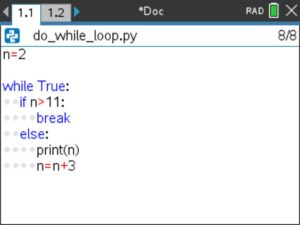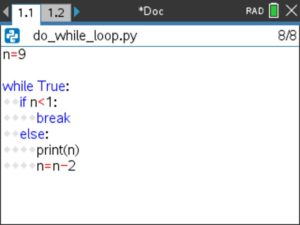## How is the Do While Loop structure different?

In light of the work we’ve looked at here with the Do While structure, we might consider the following questions:

• How is the Do While loop structure different than the other loops we’ve studied on the TI-Nspire™ CX II graphing calculator (specifically, the For loop and the more general case of the While loop)?
• What role do If conditional statements play in this Do While loop structure?

Note that the Do While loop structure is always processed at least once, since it doesn’t have a condition that is checked prior to entry into the loop (here, we model this with a While loop where the initial condition is set to True). This is quite different than the For and (more general) While loops we studied in the workshop, as these loops have conditions that are checked before the loop is ever entered into (if at all).

Since there isn’t an initial condition that is checked for a Do While loop, the programmer must build a break command into the loop, or the loop will act as an infinite loop (running again and again and again …). An If conditional statement houses this break command to end the loop. The condition on the If statement determines whether the loop will run again or whether the loop will exit.

Note that this loop structure is slightly different than Do While loop structures that appear in other programming languages. Python doesn’t have a built-in Do While loop structure, but the behavior of a Do While loop can be modeled with programs that use While, If and break commands as we’re exploring here.

## Formalizing Do While loops

Let’s use an interactive notes page to formalize the Do While loop behavior that these structures model.

Before we do that, take a minute to trace through the program outlined in the screenshot below. What do you think the output of this program will be if the program is executed with a method call of multiples(50)? Take a minute to trace through the loop and write down your prediction.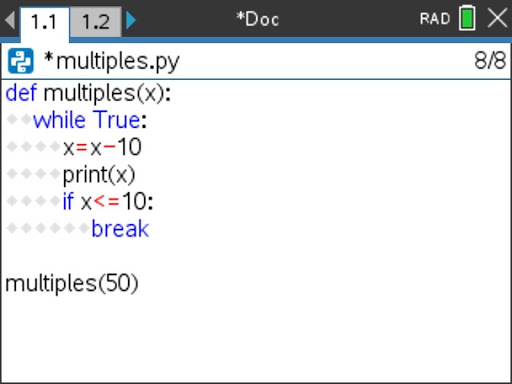Here are two pictures of the Do While Loops notes page designed to capture our important learnings about Do While loops. This notes page uses the program multiples(x) that you just traced through above.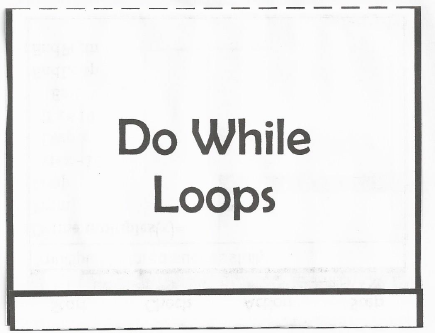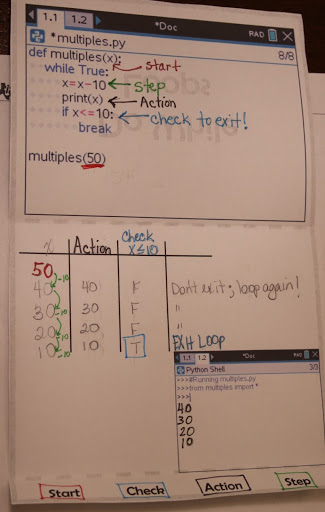How does the output shown in the notes above match with your earlier prediction?

In this notes page, we’ve used a table to trace through the loop, noting the value of x, actions taken within the loop, and the check that is run during each iteration of the loop. Tracing a loop with a table might seem unnecessary in a loop such as the one shown here. However, this is an important strategy that can be very helpful when tracing increasingly complex loops.

Also note the identification of the four parts of the loop: the start of the loop (or initial value of x), the check (to see if the loop will exit or run again), the action (within the body of the loop), and the step (to increment x to its next value, moving closer and closer to the loop eventually exiting).

Lastly, consider how different initial values of x would have affected the output of this program. What if we had executed the program with a method call of multiples(0)? In this case, the initial value of x would have already been less than 10 (the If condition that leads to the break of the loop). However, since this condition isn’t checked until after the loop has already been entered (and, specifically, after the step and action commands in this particular loop), the loop would still run one time and would display an output of -10 before the loop exited. (Do you see why?) This is a great example of the fact that post-test loops (such as Do While loops, modeled here with a While loop) always run at least one time.

## Reflecting on Do While loops

In this activity, we explored Do While Loops, a form of post-test loop that always runs at least one time.

Consider:

• When might we use a While loop with a built-in break command? Why?
• When might the fact that a post-test loops always executes at least once be helpful?

The next activity in this series will consider all three loop structures (For, While, and Loop) in contrast to each other. We will explore how the Do While loop structure used here is similar to and different than a For loop and a While loop. Additionally, we will explore the use of counters and accumulators.

This series includes:

Mac is a trademark of Apple Inc., registered in the U.S. and other countries. Windows is a registered trademark of its owner.